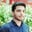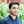Trusted answers to developer questions
Trusted Answers to Developer Questions

Related Tags

dataframe
rmod
pandas
python
communitycreator

# What is Python DataFrame.rmod() in pandas?Salman Yousaf

Grokking Modern System Design Interview for Engineers & Managers

Ace your System Design Interview and take your career to the next level. Learn to handle the design of applications like Netflix, Quora, Facebook, Uber, and many more in a 45-min interview. Learn the RESHADED framework for architecting web-scale applications by determining requirements, constraints, and assumptions before diving into a step-by-step design process.

pandas is a Python library used for data analysis and manipulation. It has a great variety of built-in functions to tackle numerical tables and time series. We can learn more about pandas by clicking here.

The DataFrame.rmod() function in pandas calculates the modulo between self and other DataFrames.

pandas

It is among flexible wrappers functions like add(), sub(), mul(), div(), mod(), and pow(). Although rmod() is equivalent to other % dataframe, it has support to fill NaN values.

### Syntax

DataFrame.rmod(other, axis='columns', level=None, fill_value=None)

### Parameters

It takes the following argument values.

• other: This can be a series, DataFrame, sequence, or scalar.
• axis='columns': {1 or 'columns', 0 or 'index'}: This determines whether to compare by column or index.
• level=None: This is the broadcast value across a level that can either be an integer or None.
• fill_value=None: This can either be a floating-point value or None.

### Return value

Pandas DataFrame: It returns the result of the arithmetic operationModulo.

### Example

In this coding example, we will create a DataFrame and a series, and then we will compute rmode between them.

# Importing Pandas as alias pd
import pandas as pd
# Creating a Pandas DataFrame
df = pd.DataFrame({"Length":[14, 33, 38, 34, 42],
"Width":[32, 21, 41, 33, 40],
"Area":[12, 14, 16, 13, 14],
"Perimeter":[4, 13, 5, 12, 9]},
index =["Shape1", "Shape2", "Shape3", "Shape4", "Shape5"])
# Creating a series
_series = pd.Series([12, 24, 128, 38], index =["Length", "Width", "Area", "Perimeter"])
# Invoking rmode()
df.rmod(_series, axis= 1)
# Printing the DataFrame
print(df)
DataFrame.rmod() implementation

### Explanation

• Lines 4–8: We create a pandas DataFrame from the argument as a dictionary.
• Line 10: We create a pandas series with the same features as the above created DataFrame.
• Line 12: We invoke df.rmode(), which calculates the modulo between each index of the DataFrame and the _series series. axis=1 means that the binary module will be calculated column-wise.
• Line 14: We print the DataFrame that contains the binary modulo at each index to the console.

RELATED TAGS

dataframe
rmod
pandas
python
communitycreator

CONTRIBUTORSalman Yousaf

Grokking Modern System Design Interview for Engineers & Managers

Ace your System Design Interview and take your career to the next level. Learn to handle the design of applications like Netflix, Quora, Facebook, Uber, and many more in a 45-min interview. Learn the RESHADED framework for architecting web-scale applications by determining requirements, constraints, and assumptions before diving into a step-by-step design process.

Keep Exploring

Learn in-demand tech skills in half the time